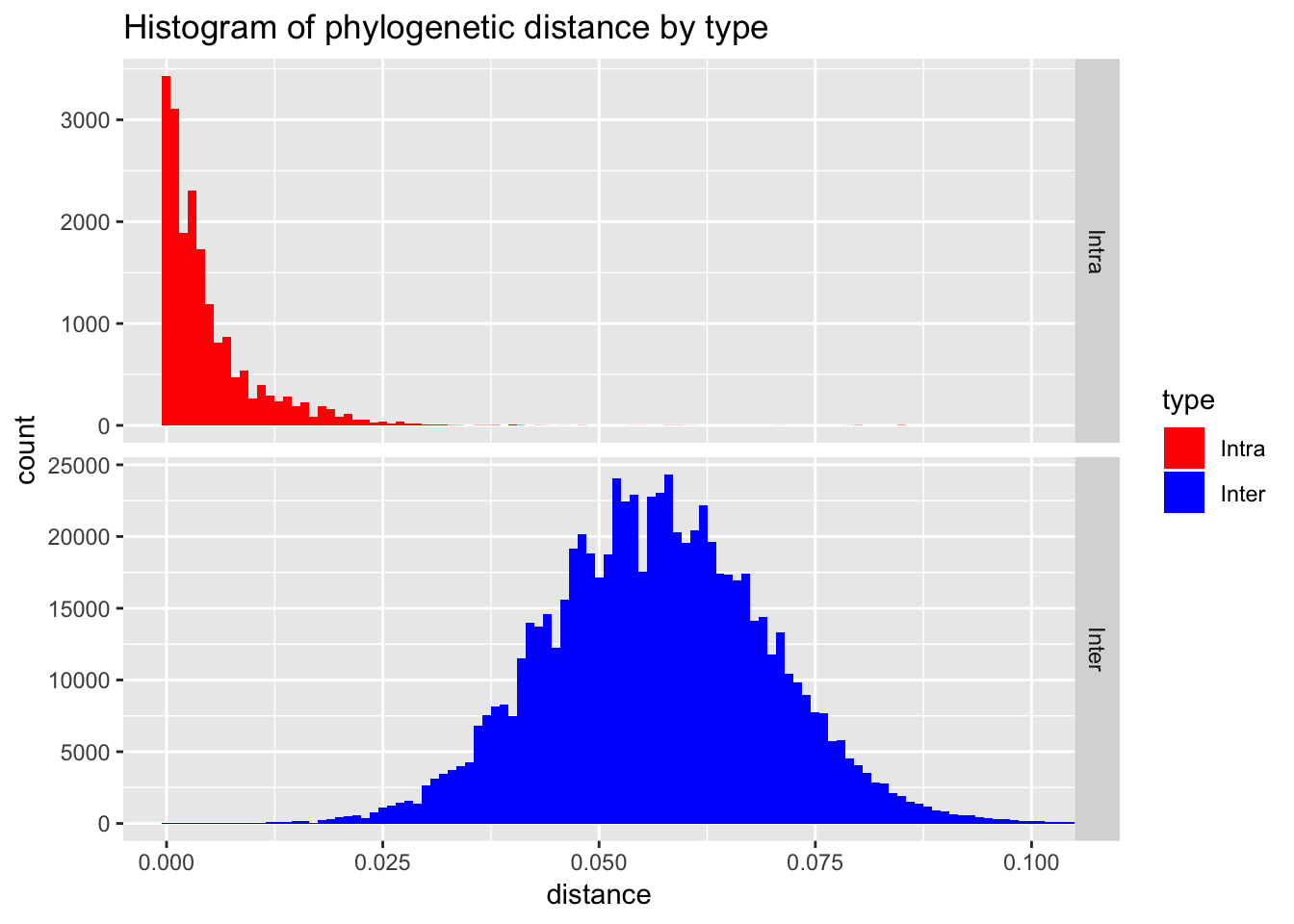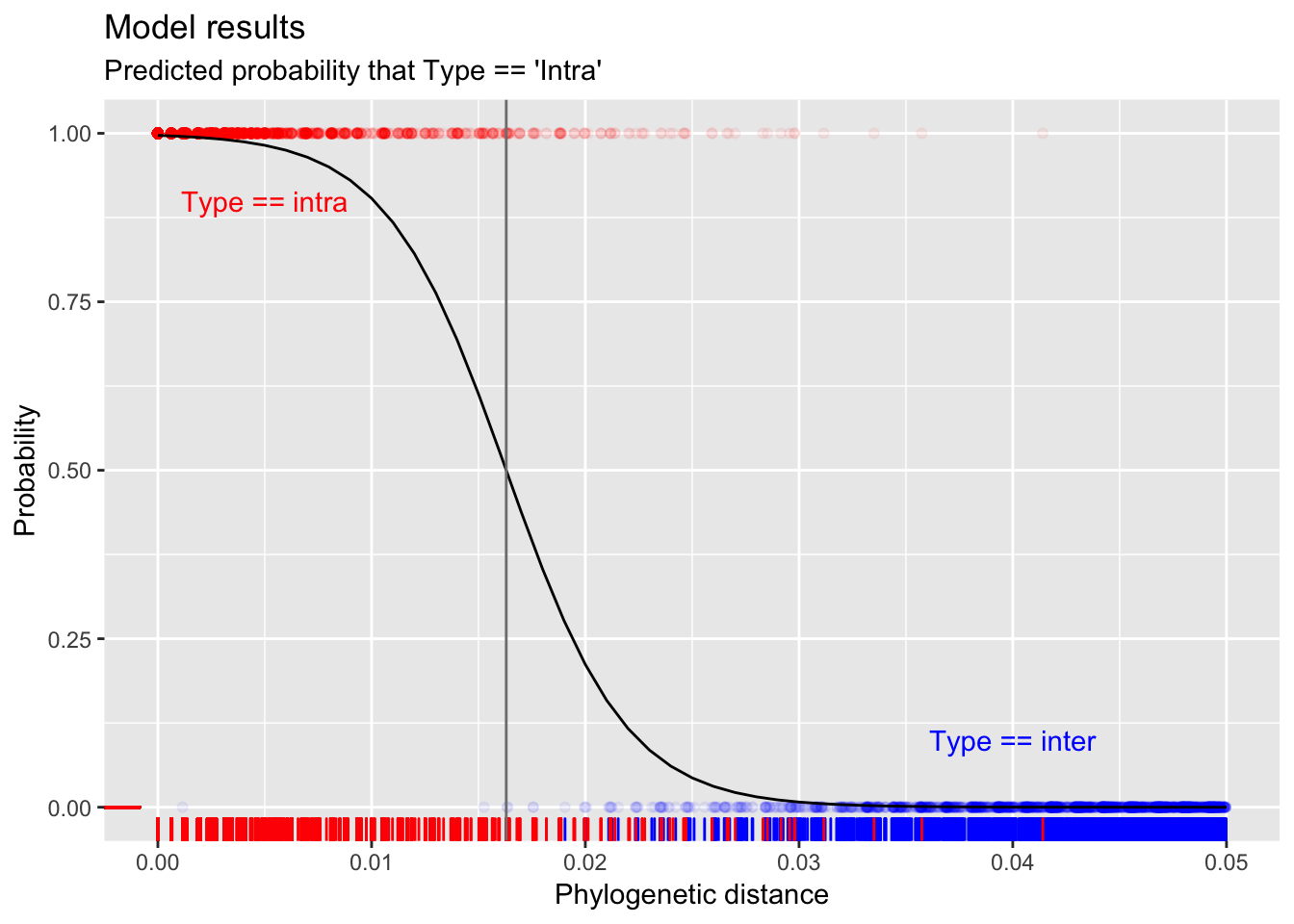# Analysing the HIV pandemic, Part 4: Classification of lab samples

by Andrie de Vries and Armand Bester

Andrie de Vries is the author of “R for Dummies” and a Solutions Engineer at RStudio

Phillip (Armand) Bester is a medical scientist, researcher, and lecturer at the Division of Virology, University of the Free State, and National Health Laboratory Service (NHLS), Bloemfontein, South Africa

In this post we complete our series on analysing the HIV pandemic in Africa. Previously we covered the bigger picture of HIV infection in Africa, and a pipeline for drug resistance testing of samples in the lab.

Then, in part 3 we saw that sometimes the same patient’s genotype must be repeatedly analysed in the lab, from samples taken years apart.

Let’s say we have genotyped a patient five years ago and we have a current genotype sequence. It should be possible to retrieve the previous sequence from a database of sequences without relying on identifiers only or at all. Sometimes when someone remarries they may change their surname or transcription errors can be made, which makes finding previous samples tedious and error-prone. So instead of using patient information to look for previous samples to include, we can rather use the sequence data itself and then confirm the sequences belong to the same patient or investigate any irregularities. If we suspect mother-to-child transmission from our analysis, we confirm this with the healthcare worker who sent the sample.

In this final part, we discuss how the inter- and intra-patient HIV genetic distances were analyzed using logistic regression to gain insights into the probability distribution of these two classes. In other words, the goal is to find a way to tell whether two genetic samples are from the same person or from two different people.

Samples from the same person can have slightly different genetic sequences, due to mutations and other errors. This is especially useful in comparing samples of genetic material from retroviruses.

## Preliminary analysis

To help answer this question, we downloaded data from the Los Alamos HIV sequence database (specifically, Virus HIV-1, subtype C, genetic region POL CDS).

Each observation is the (dis)similarity distance between different samples.

library(readr)
library(dplyr)
library(ggplot2)
## Warning: package 'ggplot2' was built under R version 3.5.2
pt_distance <-

head(pt_distance)
## # A tibble: 6 x 6
##   sample1                sample2                 distance sub   area  type
##   <chr>                  <chr>                      <dbl> <chr> <chr> <fct>
## 1 KI_797.67744.AB874124… KI_481.67593.AB873933.…   0.0644 B     INT   Inter
## 2 502-2794.39696.JF3202… WC3.27170.EF175209.B.U…   0.0418 B     INT   Inter
## 3 KI_882.67653.AB874186… KI_813.67589.AB874131.…   0.0347 B     INT   Inter
## 4 HTM360.13332.DQ322231… C11-2069070.63977.AB87…   0.0487 B     INT   Inter
## 5 O5598.34737.GQ372062.… LM49.4011.AF086817.B.T…   0.0360 B     INT   Inter
## 6 GKN.45901.HQ026515.B.… C11-2069083.65198.AB87…   0.0699 B     INT   Inter

Next, plot a histogram of the distance between samples. This clearly shows that the distance between samples of the same subject (intra-patient) is smaller than the distance between different subjects (inter-patient). This is not surprising.

However, from the histogram it is also clear that there is not a clear demarcation between these types. Simply eye-balling the data seems to indicate that one could use an arbitrary threshold of around 0.025 to indicate whether the sample is from the same person or different people.

pt_distance %>%
mutate(
type = forcats::fct_rev(type)
) %>%
ggplot(aes(x = distance, fill = type)) +
geom_histogram(binwidth = 0.001) +
facet_grid(rows = vars(type), scales = "free_y") +
scale_fill_manual(values = c("red", "blue")) +
coord_cartesian(xlim = c(0, 0.1)) +
ggtitle("Histogram of phylogenetic distance by type")## Modeling

Since we have two sample types (intra-patient vs inter-patient), this is a binary classification problem.

Logistic regression is a simple algorithm for binary classification, and a special case of a generalized linear model (GLM). In R, you can use the glm() function to fit a GLM, and to specify a logistic regression, use the family = binomial argument.

In this case we want to train a model with distance as independent variable, and type the dependent variable, i.e. type ~ distance.

We train on 100,000 (n = 1e5) observations purely to reduce computation time:

pt_sample <-
pt_distance %>%
sample_n(1e5)
model <- glm(type ~ distance, data = pt_sample, family = binomial)
## Warning: glm.fit: fitted probabilities numerically 0 or 1 occurred

(Note that sometimes the model throws a warning indicating numerical problems. This happens because the overlap between intra and inter is very small. If there is a very sharp dividing line between classes, the logistic regression algorithm has problems to converge.)

However, in this case the numerical problems doesn’t actually cause a practical problem with model itself.

The model summary tells us that the distance variable is highly significant (indicated by the ***):

summary(model)
##
## Call:
## glm(formula = type ~ distance, family = binomial, data = pt_sample)
##
## Deviance Residuals:
##     Min       1Q   Median       3Q      Max
## -3.4035  -0.0050  -0.0010  -0.0002   8.4904
##
## Coefficients:
##              Estimate Std. Error z value Pr(>|z|)
## (Intercept)    5.7887     0.1796   32.23   <2e-16 ***
## distance    -355.1454     9.3247  -38.09   <2e-16 ***
## ---
## Signif. codes:  0 '***' 0.001 '**' 0.01 '*' 0.05 '.' 0.1 ' ' 1
##
## (Dispersion parameter for binomial family taken to be 1)
##
##     Null deviance: 23659.2  on 99999  degrees of freedom
## Residual deviance:  1440.5  on 99998  degrees of freedom
## AIC: 1444.5
##
## Number of Fisher Scoring iterations: 12

Now we can use the model to compute a prediction for a range of genetic distances (from 0 to 0.05) and create a plot.

newdata <-  data.frame(distance = seq(0, 0.05, by = 0.001))
pred <- predict(model, newdata, type = "response")
plot_inter <-
pt_sample %>%
filter(distance <= 0.05, type == "Inter") %>%
sample_n(2000)

plot_intra <-
pt_sample %>%
filter(distance <= 0.05, type == "Intra") %>%
sample_n(2000)

threshold <-  with(newdata, approx(pred, distance, xout = 0.5))\$y

ggplot() +
geom_point(data = plot_inter, aes(x = distance, y = 0), alpha = 0.05, col = "blue") +
geom_point(data = plot_intra, aes(x = distance, y = 1), alpha = 0.05, col = "red") +
geom_rug(data = plot_inter, aes(x = distance, y = 0), col = "blue") +
geom_rug(data = plot_intra, aes(x = distance, y = 0), col = "red") +
geom_line(data = newdata, aes(x = distance, y = pred)) +
annotate(x = 0.005, y = 0.9, label = "Type == intra", geom = "text", col = "red") +
annotate(x = 0.04, y = 0.1, label = "Type == inter", geom = "text", col = "blue") +
geom_vline(xintercept = threshold, col = "grey50") +
ggtitle("Model results", subtitle = "Predicted probability that Type == 'Intra'") +
xlab("Phylogenetic distance") +
ylab("Probability")Logistic regression essentially fits an s-curve that indicates the probability. In this case, for small distances (lower than ~0.01) the probability of being the same person (i.e., type is intra) is almost 100%. For distances greater than 0.03 the probability of being type intra is almost zero (i.e., the model predicts type inter).

The model puts the distance threshold at approximately 0.016.

## The practical value of this work

In part 2, we discussed how researchers developed an automated pipeline of phylogenetic analysis. The project was designed to run on the Raspberry Pi, a very low-cost computing device. This meant that the cost of implementation of the project is low, and the project has been implemented at the National Health Laboratory Service (NHLS) in South Africa.

In this part, we described the very simple logistic regression model that runs as part of the pipeline. In addition to the descriptive analysis, e.g., heat maps and trees (as described in part 3), this logistic regression makes a prediction whether two samples were obtained from the same person, or from two different people. This prediction is helpful in allowing the laboratory staff identify potential contamination of samples, or indeed to match samples from people who weren’t matched properly by their name and other identifying information (e.g., through spelling mistakes or name changes).

Finally, it’s interesting to note that traditionally the decision whether two samples were intra-patient or inter-patient was made on heuristics, instead of modelling. For example, a heuristic might say that if the genetic distance between two samples is less than 0.01, they should be considered a match from a single person.

Heuristics are easy to implement in the lab, but sometimes it can happen that the origin of the original heuristic gets lost. This means that it’s possible that the heuristic is no longer applicable to the sample population.

This modelling gave the researchers a tool to establish confidence intervals around predictions. In addition, it is now possible to repeat the model for many different local sample populations of interest, and thus have a tool that is better able to discriminate given the most recent data.

## Conclusion

In this multi-part series of HIV in Africa we covered four topics:

• In part 1, we analysed the incidence of HIV in sub-Sahara Africa, with special mention of the effect of the wide-spread availability of anti-retroviral (ARV) drugs during 2004. Since then, there was a rapid decline in HIV infection rates in South Africa.
• In part 2, we described the PhyloPi project - a phylogenetic pipeline to analyse HIV in the lab, available for the low-cost RaspBerry Pi. This work as published in the PLoS ONE journal: “PhyloPi: An affordable, purpose built phylogenetic pipeline for the HIV drug resistance testing facility
• Then, part 3 described the biological mechanism how the HIV virus mutates, and how this can be modeled using a Markov chain, and visualized as heat maps and phylogenetic trees.
• This final part covered how we used a very simple logistic regression model to identify if two samples in the lab came from the same person or two different people.

## Closing thoughts

I hope that you enjoyed this series on ‘Analysing the HIV pandemic’ using R and some of the tools available as part of the tidyverse packages. Learning R provided me not only with a tool set to analyse data problems, but also a community. Being a biologist, I was not sure of the best approach for solving the problem of inter- and intra-patient genetic distances. I contacted Andrie from Rstudio, and not only did he help us with this, but he was also excited about it. It was a pleasure telling you about our journey on this blog site, and a privilege doing this with experts.